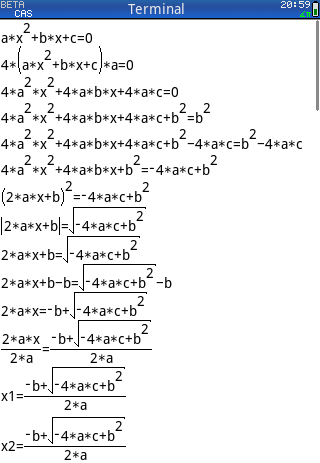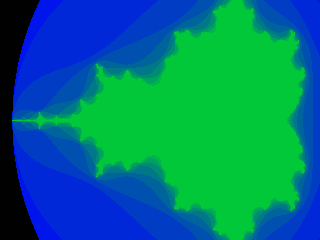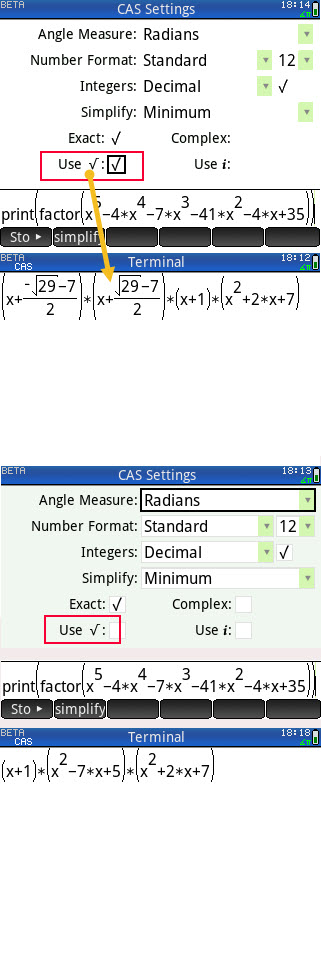2021: Examples of CAS-type programs
05-01-2021, 12:22 AM (This post was last modified: 05-09-2021 11:25 PM by compsystems.)
Post: #1compsystemsSenior Member Posts: 1,342 Joined: Dec 2013
2021: Examples of CAS-type programs
Hello

In this post I am going to put a series of examples of CAS-type programs

SCRIPT 01: obtaining the solutions of the quadratic equation

You can run the following sentences line by line in the CAS history, please let me know if the results on your calculator are different.

Change manually Simplify mode to None [shift] + [CAS (settings)]
autosimplify cmd of Xcas is not yet available in firmware 2021
autosimplify (0); // Xcas only = (SCRIPT 01
PHP Code:
    autosimplify(0); // Xcas only =(    purge(a,b,c,x,equ0,equ1,equ2);    equ0:= (a*x^2+b*x+c) = 0; // [enter]  (a*x^2+b*x+c) = 0    equ := equ0 * 4*a;        // [enter]  (4*(a*x^2+b*x+c)*a) = 0    equ := expand( equ );    // [enter]  (4*a^2*x^2+4*a*b*x+4*a*c) = 0    equ := equ + b^2;        // [enter]  (4*a^2*x^2+4*a*b*x+4*a*c+b^2) = (b^2)    equ := equ - 4*a*c;      // [enter]  (4*a^2*x^2+4*a*b*x+4*a*c+b^2-4*a*c) = (b^2-4*a*c)    equ := simplify( equ );  // [enter]  (4*a^2*x^2+4*a*b*x+b^2) = (-4*a*c+b^2)    equ := factor( equ );    // [enter]  ((2*a*x+b)^2) = (-4*a*c+b^2)    equ := √( equ );         // [enter]   (ABS(2*a*x+b)) = (sqrt(-4*a*c+b^2))    equ := expr( replace( string( equ ), "abs", "" ) ); // [enter]  (2*a*x+b) = (sqrt(-4*a*c+b^2))    equ := equ - b;          // [enter]   (2*a*x+b-b) = (sqrt(-4*a*c+b^2)-b)    equ := simplify( equ );  // [enter]   (2*a*x) = (-b+sqrt(-4*a*c+b^2))    equ := equ / ( 2*a );    // [enter]   (2*a*x/(2*a)) = (-b+sqrt(-4*a*c+b^2))/(2*a)    equ := simplify( equ );  // [enter]   x = (-b+sqrt(-4*a*c+b^2))/(2*a)    equ1 := expr( replace( string( equ ), "x", "x1" ) );    // [enter]   x1 = (-b+sqrt(-4*a*c+b^2))/(2*a)    equ2 := expr( replace( string( equ1 ), "x1", "x2" ));    // [enter]  x2 = (-b+sqrt(-4*a*c+b^2))/(2*a)    equ2 := expr( replace( string( equ2 ), "-b+", "-b-" ) ); // [enter]  x2 = (-b-sqrt(-4*a*c+b^2))/(2*a)

Please inform me if I am wrong.
What other calculator with CAS and original firmware can do the above? I think no one, only the HP-Prime with Xcas, simply because they (TI; Texas Instruments, CASIO, Sharp, Numworks ...) can't control the simplification type of output.

the same script above but with comments

PHP Code:
    autosimplify(0);     purge(a,b,c,x,equ0,equ1,equ2);    equ0:= (a*x^2+b*x+c) = 0;    equ := equ0 * 4*a;    equ := expand( equ );    equ := equ + b^2;    equ := equ - 4*a*c;    equ := simplify( equ );    equ := factor( equ );    equ := √( equ );    equ := expr( replace( string( equ ), "abs", "" ) );    equ := equ - b;    equ := simplify( equ );    equ := equ / ( 2*a );    equ := simplify( equ );    equ1 := expr( replace( string( equ ), "x", "x1" ) );    equ2 := expr( replace( string( equ1 ), "x1", "x2" ));    equ2 := expr( replace( string( equ2 ), "-b+", "-b-" ) );

the same script above but using SUBST to make changes to the expression without converting to text strings

PHP Code:
    autosimplify(0);     purge(a,b,c,x,equ0,equ1,equ2);    equ0:= (a*x^2+b*x+c) = 0;    equ := equ0 * 4*a;     equ := expand( equ );    equ := equ + b^2;    equ := equ - 4*a*c;    equ := simplify( equ );    equ := factor( equ );    equ := √( equ );    equ := subst(equ,'abs', nop);    equ := equ - b;     equ := simplify( equ );    equ := equ / ( 2*a );    equ := simplify( equ );     equ1 := subst(equ,x, x1); // or, equ1 := subst(equ,x=x1);    equ2 := subst(equ1,x1, x2); // or, equ2 := subst(equ1,x1=x2);    equ2 := subst(equ1,"-b+", "-b+");

as a program and 2D printing

PHP Code:
#cas//Deduction Quadratic Formulaquadraticform():=begin     autosimplify(0);    purge(a,b,c,x,equ0,equ1,equ2);    equ0:= (a*x^2+b*x+c) = 0;         print(equ0);    equ := equ0 * 4*a;                print(equ);     equ := expand( equ );             print(equ);    equ := equ + b^2;                 print(equ);    equ := equ - 4*a*c;               print(equ);    equ := simplify( equ );           print(equ);    equ := factor( equ );             print(equ);    equ := √( equ );                  print(equ);    equ := subst(equ,'abs', nop);     print(equ);    equ := equ - b;                   print(equ);     equ := simplify( equ );           print(equ);    equ := equ / ( 2*a );             print(equ);    equ := simplify( equ );                equ1 := subst(equ,x=x1);          print(equ1);    equ2 := subst(equ1,x1=x2);            equ2 := subst(equ2,"-b+", "-b+"); print(equ2);    return [equ0, equ1, equ2];end;Printing with the #183 character "·" would look more compact and legible, this will allow to display the "*" symbol as a "·", this could be changed by configuration, depending on how you want to display the multiplication operator. This feature was available on the hp48gx

using optional template for cas prg
PHP Code:
#casfunction name(args)   ...   return ...end;#end

Code:
#cas //Deduction Quadratic Formula function quadraticform()     autosimplify(0);     purge(a,b,c,x,equ0,equ1,equ2);     equ0:= (a*x^2+b*x+c) = 0;         print(equ0);     equ := equ0 * 4*a;                print(equ);      equ := expand( equ );             print(equ);     equ := equ + b^2;                 print(equ);     equ := equ - 4*a*c;               print(equ);     equ := simplify( equ );           print(equ);     equ := factor( equ );             print(equ);     equ := √( equ );                  print(equ);     equ := subst(equ,'abs', nop);     print(equ);     equ := equ - b;                   print(equ);      equ := simplify( equ );           print(equ);     equ := equ / ( 2*a );             print(equ);     equ := simplify( equ );                 equ1 := subst(equ,x=x1);          print(equ1);     equ2 := subst(equ1,x1=x2);             equ2 := subst(equ2,"-b+", "-b+"); print(equ2);     return [equ0, equ1, equ2]; end;

Script using only the ANS variable
PHP Code:
    (a*x^2+b*x+c) = 0;     Ans*4*a;          expand(Ans);        Ans+b^2;          Ans-4*a*c;        simplify(Ans);     factor(Ans);       √(Ans);           subst(Ans,'abs', nop);     Ans-b;              simplify(Ans);    Ans/(2*a);        simplify(Ans);      expr(replace(string(Ans),"-b+","-b-"));as prgm
PHP Code:
#cas//Deduction Quadratic Formulafunction quadraticform_1()    (a*x^2+b*x+c) = 0;     Ans*4*a;          expand(Ans);        Ans+b^2;          Ans-4*a*c;        simplify(Ans);     factor(Ans);       √(Ans);           subst(Ans,'abs', nop);     Ans-b;              simplify(Ans);    Ans/(2*a);        simplify(Ans);      expr(replace(string(Ans),"-b+","-b-"));    return Ans;end;

in writing.
05-01-2021, 07:20 AM
Post: #2
 jonmooreMember Posts: 284 Joined: Apr 2020
RE: 2021 Examples of CAS-type programs
When using KhiCAS all of the following calculators can match this workflow.

- NI Nspire CX II (CAS and non-CAS models)
- Numworks
- Casio CG50 and the low-cost Casio FX-9860G-III

Bernards push to provide a universal CAS for a wide range of hardware inclusive of exam friendly non-CAS models is an interesting challenge for HP to answer. Not to sound like a scratched record, I think it's time that HP allows developers like Bernard to provide solutions coded in C to run directly on the Prime. As Bernard himself has stated, Casio allows developers to provide solutions of this nature natively and it has no effect on the perception of Casio calculators as being exam friendly. NI of course forces the community to use Ndlesss, but these days it's a matter of days between NI updating the firmware and Ndless providing a matching solution.

Whilst it's true to say that the HP Prime G2 is the most powerful calculator to process programs of the nature detailed in post 1 (and with more available RAM); all of the competitor calculators mentioned above are fast enough these days that the average user won't notice any difference. There's even a chance that the NI Nspire CX II will be faster as KhiCAS is running directly on the ARM hardware, not via a host OS.

None of what I write above should be taken as me preferring any of the aforementioned competitor calculators, but it is important that a balanced view is acknowledged.
05-01-2021, 01:16 PM
Post: #3
 rprosperiSuper Moderator Posts: 5,646 Joined: Dec 2013
RE: 2021 Examples of CAS-type programs
NI ?

Is there some reason to not call them TI ?

--Bob Prosperi
05-01-2021, 06:08 PM
Post: #4
 roadrunnerSenior Member Posts: 429 Joined: Jun 2015
RE: 2021 Examples of CAS-type programs
Perhaps jonmoore is one of the Knights who say NI?

https://en.wikipedia.org/wiki/Knights_Who_Say_%22Ni!%22

05-01-2021, 06:39 PM
Post: #5
 jonmooreMember Posts: 284 Joined: Apr 2020
RE: 2021 Examples of CAS-type programs
(05-01-2021 06:08 PM)roadrunner Wrote:  Perhaps jonmoore is one of the Knights who say NI?

https://en.wikipedia.org/wiki/Knights_Who_Say_%22Ni!%22What a random slip! The NI acronym I use all the time is Native Instruments (I own the whole kaboodle of their kit) as I have a music production setup.

https://www.native-instruments.com/en/
05-01-2021, 08:27 PM
Post: #6
 rprosperiSuper Moderator Posts: 5,646 Joined: Dec 2013
RE: 2021 Examples of CAS-type programs
(05-01-2021 06:08 PM)roadrunner Wrote:  Perhaps jonmoore is one of the Knights who say NI?

https://en.wikipedia.org/wiki/Knights_Who_Say_%22Ni!%22

I originally wrote something along these lines, but then thought it too obscure and removed the reference to the Knights.

Thanks for restoring my faith that this is a universal reference.--Bob Prosperi
05-02-2021, 11:29 AM
Post: #7
 jonmooreMember Posts: 284 Joined: Apr 2020
RE: 2021 Examples of CAS-type programs
(05-01-2021 08:27 PM)rprosperi Wrote:
(05-01-2021 06:08 PM)roadrunner Wrote:  Perhaps jonmoore is one of the Knights who say NI?

https://en.wikipedia.org/wiki/Knights_Who_Say_%22Ni!%22

I originally wrote something along these lines, but then thought it too obscure and removed the reference to the Knights.

Thanks for restoring my faith that this is a universal reference.@TheRealPython, too obscure - maybe in an alternative universe!BTW @roadrunner scored double points for the aptness of their punnery!
05-02-2021, 05:26 PM
Post: #8BruceHSenior Member Posts: 434 Joined: Dec 2013
RE: 2021 Examples of CAS-type programs
(05-01-2021 12:22 AM)compsystems Wrote:  What other calculator with CAS and original firmware can do the above?

The 28C had a menu that allowed manipulations of sub-parts of an expression that got lost in the transition to the 48SX.

Although not nearly as sophisticated as your examples here, it allowed things like selecting part of an expression and adding zero or multiplying by 1. This then made it easier to re-arrange things by moving parts to the other side of the equals sign.

Clearly, back in the days of the 28C such things were much quicker to do by hand but I always wondered why it got dropped - it's an educational tool after all - and your post brought it all back.
05-02-2021, 07:01 PM
Post: #9
 jonmooreMember Posts: 284 Joined: Apr 2020
RE: 2021 Examples of CAS-type programs
Just spotted the original firmware edit to the original post. If you're going to edit your original post because the facts were wrong at least have the good grace to acknowledge the reasons why...

I'm a huge fan of HP calculators but I really can't be dealing with tribalism. The original unedited post was factually incorrect, hence my follow up post. This post wasn't intended to dis the Prime, it's clearly the best hardware in its competitor set. But Bernards belief in making CAS technology freely available to all possible OS environments, inclusive of a variety of non-HP calculators is to be applauded.

It doesn't necessarily make KhiCAS better than the XCAS integration on the Prime. In fact, the key word here is integration. Whilst KhiCAS on the TI Nspire CX II is to all intents and purposes the full desktop version of XCAS, the fact that it's a separate application that's not integrated into the host OS means that it will never be a match for the HP Prime in terms of 'calculator UX' flexibility. But it has its own UX strengths too.
05-02-2021, 07:19 PM
Post: #10compsystemsSenior Member Posts: 1,342 Joined: Dec 2013
RE: 2021 Examples of CAS-type programs
Hello
With or without "original firmware" is the same, because third-party applications that are not factory integrated do not count.
But assuming third party applications, HP-Prime CAS is far inferior to KhiCAS as it does not include all the functions that can be executed within the calculator hardware.
I do not even understand what is the difficulty for hpprime CAS to include almost all the Xcas functions, Bernard said that it is not him who decides which functions are made visible or not. This implies that the functions are hidden or could be included very easily.

I hope that the hp-prime development group will reconsider it and give us the possibility to have almost all the Xcas functions in the hp-prime, they do not lose anything, on the contrary we all win.
Or why don't they want to include the full CAS? What limits this inclusion RAM, hardware?

Only in the first example I show that we need the autosimplify() function since when redistributing a code I have to tell the user, manually he has to modify some flags, but within a code I need to automatically change the flags and in this case it is no longer feasible to tell user to change x flags again.

Remember that I use a translator, please let me know if any word sounds bad
05-02-2021, 09:17 PM
Post: #11
 jonmooreMember Posts: 284 Joined: Apr 2020
RE: 2021 Examples of CAS-type programs
(05-02-2021 07:19 PM)compsystems Wrote:  Remember that I use a translator, please let me know if any word sounds bad

All good.05-06-2021, 03:59 AM (This post was last modified: 05-10-2021 03:11 AM by compsystems.)
Post: #12compsystemsSenior Member Posts: 1,342 Joined: Dec 2013
RE: 2021 Examples of CAS-type programs
cas + pixels cmds

Example with standard CAS notation.
PHP Code:
#casfract1( X_, Y_, Nmax ):=begin        // Mandelbrot fractal, not using symmetry.    local x, y, z, c, j, w, h, res1;    //freeze;    w := 2.7/X_;    h := -1.87/Y_;    res1 := [];     Y_ := Y_-1;    for y from 0 to Y_ do        c := -2.1 + i*(h*y+0.935);        for x from 0 to X_-1 do            z := 0;            for j from 0 to Nmax-1 do                if abs( z := z^2+c )>2 then break; end;            end;            res1.append( pixon_p( x, y, 126*j+2079 ));             c := c+w;        end;    end;    wait(0);     return "Done"; //return res1; end;#end
fract1( 320, 240, 10 ); [enter]As function xcas compatibility
PHP Code:
#casfunction fract2( X_, Y_, Nmax )        // Mandelbrot fractal, not using symmetry.    local x, y, z, c, j, w, h, res1;    w := 2.7/X_;    h := -1.87/Y_;    res1 := [];     Y_ := Y_-1;    for y from 0 to Y_ do        c := -2.1 + i*(h*y+0.935);        for x from 0 to X_-1 do            z := 0;            for j from 0 to Nmax-1 do                if abs( z := z^2+c )>2 then break; end;            end;            res1.append( pixon_p( x, y, 126*j+2079 )); // Xcas pixon_p -> pixon            c := c+w;        end;    end;    wait(0); // Xcas: comment on this line    return "Done" ;end;#end
fract2( 320, 240, 10 ); [enter]
deltalist(time(fract2(320,240,10))); // only for Xcas to determine the execution time

using symmetry
PHP Code:
#casfunction fract2a( X_, Y_, Nmax )          // Mandelbrot fractal, using symmetry.          local x, y, z, c, j, w, h, res1, res2;    //freeze;    w := 2.7/X_;    h := -1.87/Y_;    res1:=makelist(-ceiling( X_*Y_/2 )-1);    res2 := res1;     Y_ := Y_-1;    for y from 0 to Y_/2 do        c := -2.1 + i*(h*y+0.935);        for x from 0 to X_-1 do            z := 0;            for j from 0 to Nmax-1 do                if abs( z := z^2+c )>2 then break; end;            end;            res1.append( pixon_p( x, y, 126*j+2079 ));             res2.append( pixon_p( x, Y_-y, 126*j+2079 ));            c := c+w;        end;    end;    wait(0);     return "Done"; //    return res1,res2;end;#end

With Python syntax.
PHP Code:
#casdef fract3( X_, Y_, Nmax ):    local x, y, z, c, j     for x in range( X_ ):        for y in range( Y_ ):            z = 0            c = 2.7*x / X_-2.1 + i*( -1.87*y/Y_ + .935)            for j in range( Nmax ):                z = z*z + c                if abs( z ) > 2:  # abs(z = z*z+c)>2:                    break            pixon_p( x, y, 255*20*j + 256 )    wait(0)    return "Done"#end
fract3( 320, 240, 10 ); [enter]XCAS only
PHP Code:
function fract2( X_, Y_, Nmax )    local x, y, z, c, j, w, h, res1;    w := 2.7/X_;    h := -1.87/Y_;    res1 := [];     Y_ := Y_-1;    for y from 0 to Y_ do        c := -2.1+i*(h*y+0.935);        for x from 0 to X_-1 do            z := 0;            for j from 0 to Nmax-1 do                if abs( z := z^2+c )>2 then break; end;            end;            res1.append( pixon( x, y, 126*j+2079 )); // hpprime pixon -> pixon_p            c := c+w;        end;    end;    return res1;end
deltalist(time(fract2(320,240,10))); [enter]

XCAS only
PHP Code:
function fract2a( X_, Y_, Nmax )      // Mandelbrot fractal, using symmetry.          local x, y, z, c, j, w, h, res1, res2;    w := 2.7/X_;    h := -1.87/Y_;    res1:=makelist(-ceiling( X_*Y_/2 )-1);    res2 := res1;     Y_ := Y_-1;    for y from 0 to Y_/2 do        c := -2.1 + i*(h*y+0.935);        for x from 0 to X_-1 do            z := 0;            for j from 0 to Nmax-1 do                if abs( z := z^2+c )>2 then break; end;            end;            res1.append( pixon( x, y, 126*j+2079 ));             res2.append( pixon( x, Y_-y, 126*j+2079 ));            c := c+w;        end;    end;    return res1,res2;end
05-07-2021, 02:26 AM (This post was last modified: 05-08-2021 03:26 PM by compsystems.)
Post: #13compsystemsSenior Member Posts: 1,342 Joined: Dec 2013
RE: 2021 Examples of CAS-type programs
"WHEN" "IFTE"and "()?" CMDS

WHEN CMD
4 arguments
1: test to solve
2: action for true
3: action for false
4: optional if you cannot solve the test

with 4 args
PHP Code:
#castest_whencmd_cas_0(testvar):=begin    return when( testvar, true, false, "other" );end;#end

test_whencmd_cas_0(eeee) > "other"

with 3 args
PHP Code:
#castest_whencmd_cas(testvar):=begin    return when( testvar, true, false );end;#end

as function
PHP Code:
#casfunction test_whencmd_cas(testvar)    return when( testvar, true, false );end;

test_whencmd_cas(true); [enter] returns true
test_whencmd_cas(false); [enter] returns false
purge(x); test_whencmd_cas(x); [enter] returns ((x)? true : false)
test_whencmd_cas(1); test_whencmd_cas(0); test_whencmd_cas(-1);
[enter] returns true, false, true

with (?) infix operator
PHP Code:
#castest_questionmarkcmd_cas(testvar):=begin    return (testvar)? true:false;end;#end

as function
PHP Code:
#casfunction test_questionmarkcmd_cas(testvar)    return (testvar)? true:false;end;

test_questionmarkcmd_cas(1); test_questionmarkcmd_cas(0); test_questionmarkcmd_cas(-1);
[enter] returns true, false, true

test_questionmarcmd_cas(x); [enter] returns ((x)? true : false)

with IFTE cmd, the test must always return a numerical value
PHP Code:
#castest_iftecmd_cas(testvar):=begin    return ifte( testvar, true, false );end;

as function
PHP Code:
#casfunction test_iftecmd_cas(testvar)    return ifte( testvar, true, false );end;

test_iftecmd_cas(1); test_iftecmd_cas(0); test_iftecmd_cas(-1);test_iftecmd_cas(true); test_iftecmd_cas(false);
[enter]
1, 0, 1, 1, 0

test_iftecmd_cas(x); [enter] IFTE(x,1,0)

ifte + := operator
ifte(true,(testvar1:=123),(testvar2:=456)); [enter] returns 123 ok [up] [up] [copy] [enter] ok
ifte(false,(testvar1:=123),(testvar2:=456)); [enter] returns 456 ok
purge(testvar1, testvar2);
ifte(true,(123=>testvar1),(456=>testvar2));

? + => operator
(true)? (123=>testvar1): (456=>testvar2); [enter] returns 123 ok,
(false)? (123=>testvar1): (456=>testvar2); [enter] returns 456 ok, but [up] [up][copy] [enter]
"sto 123:testvar4 not allowed! Error: Bad Argument Type"
Cause of the problem: the parentheses are suppressed in the arguments (123=>testvar3) to 123=>testvar3, this means that it cannot be interpreted
05-09-2021, 10:21 PM (This post was last modified: 06-21-2021 09:07 PM by compsystems.)
Post: #14compsystemsSenior Member Posts: 1,342 Joined: Dec 2013
RE: 2021: Examples of CAS-type programs
Hello
trying the following code I have problems

PHP Code:
#castest_withsqrt_flag():=begin  local poly1;  purge(x); PRINT(); print:  poly1 := x^5 -4*x^4 -7*x^3 -41*x^2 -4*x+35;  print( factor( poly1 ) ); // (x+1) * (x^2-7*x+5) * (x^2+2*x+7) // or (x+(-√29-7)/2) * (x+(√29-7)/2)*(x+1) * (x^2+2*x+7) // depending on the square root flag it is activated or not manually.  return poly1;end;
test_withsqrt_flag() [enter] "Error: Bad Argument Value". Why?
do you have this problem on your calculator?

Now I want in the hp-prime to automate the factoring output without asking the user to change the flag manually

Xcas Code
PHP Code:
test_withsqrt_flag(x):={  local poly1;  purge(x);  with_sqrt( False );   poly1 := x^5 -4*x^4 -7*x^3 -41*x^2 -4*x+35;  print( factor( poly1 ) ); // (x+1) * (x^2-7*x+5) * (x^2+2*x+7)  with_sqrt( True );    print( factor( poly1 )); // (x+(-√29-7)/2) * (x+(√29-7)/2)*(x+1) * (x^2+2*x+7)   return poly1;};« Next Oldest | Next Newest »

User(s) browsing this thread: 1 Guest(s)